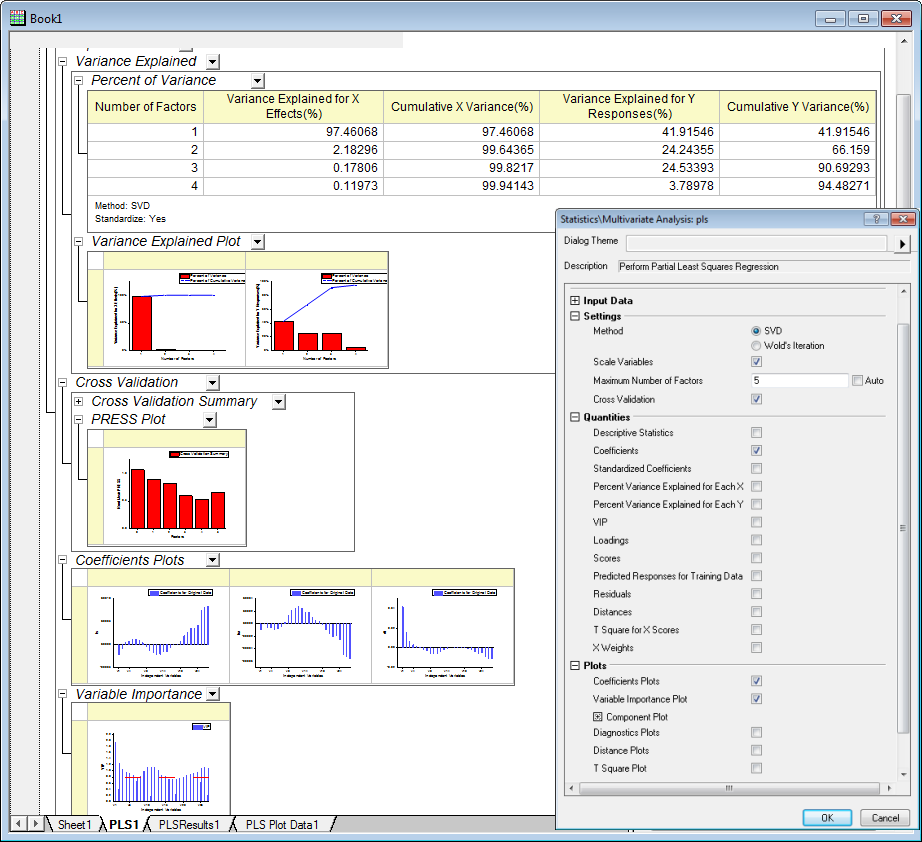# Partial Least Squares Regression (Pro)

(ORG-5412)

Partial least squares (PLS) is a method for constructing predictive models when there are many factors and they are highly collinear. It is useful for variable selection and dimension reduction

There are two primary reason for using PLS

• Prediction

PLS is most commonly used for constructing predictive model when the the information contained in a large number of original variables and they are highly collinear.

• Interpretation

PLS can be used to discover important features of a large data set. It often reveals relationships that were previously unsuspected, thereby allowing interpretations of the data that may not ordinarily result from examination of the data.

To perform partial least squares in Origin, select Statistics: Multivariate Analysis: Partial Least Square

### How to

The data in the example are reported in Umetrics (1995); the original source is Lindberg, Persson, and Wold (1983). Suppose that the scientist is researching pollution in the Baltic Sea, and they would like to use the spectra of samples of sea water to determine the amounts of three compounds present in samples from the Baltic Sea: lignin sulfonate (pulp industry pollution), humic acids (natural forest products), and detergent (optical whitener).

The scientist also has data of the spectra emission intensities at different frequencies in sample spectrum (v1-v27)

The three compounds of interest are: lignin sulfonate (ls), which is pulp industry pollution; humic acid (ha), a natural forest product; and an optical whitener from detergent (dt).

Partial least square regression can help to establish a model to predict the amounts of three compounds from v1-v27

To Perform the Analysis

1. Activate Sheet1 in Book1. Select Statistics: Multivariate Analysis: Partial Least Square  to open the Partial Least Square dialog
2. In the opened dialog, set column v1 ~ v27 as Independent Variables, column ls, ha, dt as Dependent Variables and set other settings as image below. And click OK buttonInterpreting Results

1> The Cross Validation table shows that the optimum number of factors to extract, based on Root Mean PRESS. From the foot note we will know the 4 factors is the optimum number of factors to extract.

2> The Variance Explained table reveals the proportion of variance explaned by each factor. In this example, the 1st factor explained 97.46068% varaince for X effects and 41.91546% variance for Y effects

3> The coefficients plots display the coefficients of the X variables for each Y preditor

4> The Variable Importance Plot display the VIP scores for each X variable. The VIP score is a measure of a variable’s importance in modeling both X and Y. If a variable has a small coefficient and a small VIP, then it is a candidate for deletion from the model (Wold,1995). A value of 0.8 is generally considered to be a small VIP (Eriksson et al, 2006) and a red line is drawn on the plot at 0.8.

5> Columns in the PLSResults1 sheet are coefficients values of the X variables for each Y predictor.